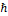### The Essence of the Elementary Charge and the Derivation and Calculation of the Electron Inertial Mass

•  Shlomo Barak

#### Abstract

We consider Electric Charge (Barak, 2019) as merely a space deformation. Positive charge is a contracted zone of space and negative charge - a dilated zone. The field of the electric charge is a smooth continuation from this zone to infinity, of a diminishing contraction or dilation of space. This consideration enables us to derive theoretically, with no phenomenology, the Maxwell theory of electrostatics. Together with the Lorentz Transformation we derive (a known derivation procedure) Maxwell’s theory in its entirety.

Our geometry of deformed zones of space (Barak, 2019), rather than Riemannian geometry of bent manifolds, enables us to attribute positive curvature to a contracted zone of space, i.e., to a positive charge, and negative curvature to a negative charge. This enables us to apply General Relativity (GR) in our derivations, and show that the positive elementary charge can be considered a kind of black hole, whereas the negative elementary charge - a white hole.

Based on the above we construct a model of the Electron (and other elementary particles) and derive and calculate its attributes, including inertial mass and spin. Neither the Standard Model nor String Theory has provided such results.

The equations for these masses contain only the constants G, c,and α (the fine structure constant). Our calculated results comply with CODATA 2014.This work is licensed under a Creative Commons Attribution 4.0 License.
• Issn(Print): 1916-9639
• Issn(Onlne): 1916-9647
• Started: 2009
• Frequency: bimonthly